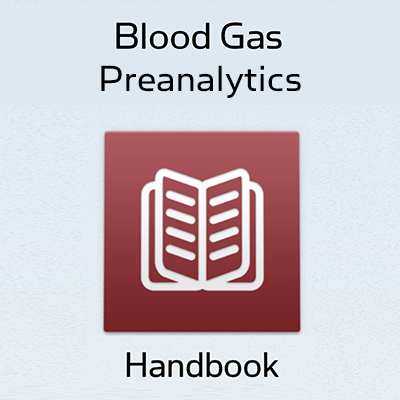Topics
Parameters

Article

March 2017

# Precision-recall curves – what are they and how are they used?

by Suzanne Ekelund
Summary This article demonstrates that adding a lot of patients without disease and with low test results to a study may improve the ROC curve significantly without any improvement in sensitivity or in positive predictive value of the parameter evaluated.

The precision-recall curves are not impacted by the addition of patients without disease and with low test results. It is highly recommended to use precision-recall curves as a supplement to the routinely used ROC curves to get the full picture when evaluating and comparing tests.

### Abbreviations and definitions

 FN False negative – number of persons with disease who have a negative test result with the assay in question. FP False positive – number of persons without disease who have a positive test result with the assay in question. FPR False positive rate – fraction of persons without disease who have a positive test result with the assay in question. Calculated as: 100 % – specificity. PPV Positive predictive value – fraction of persons with a positive test result who do have the disease. Calculated as TP / (TP + FP). PRC Precision-recall curve. A plot of precision (= PPV) vs. recall (= sensitivity) for all potential cut-offs for a test. Precision Identical to PPV. Prevalence Fraction of persons with disease in the tested population. Recall Identical to sensitivity. ROC curve Receiver operating characteristics curve. A plot of true positive fraction (= sensitivity) vs. false positive fraction (= 1 – specificity) for all potential cut-offs for a test. Sensitivity Fraction of persons with disease who get a positive test result with the assay in question. Calculated as TP / (TP + FN). Specificity Fraction of persons without disease who get a negative test result with the assay in question. Calculated as TN / (TN + FP). TN True negative – number of persons without disease who have a negative test result with the assay in question. TP True positive – number of persons with disease who have a positive test result with the assay in question. TPR True positive rate. Identical to sensitivity.

### Background

In most situations when biochemical tests are performance evaluated, the data obtained is heavily skewed or imbalanced, i.e. most subjects tested belong to the group of patients who do not have the disease/condition tested for. Typical disease prevalences are in the range of ~10 %. It means that only ~10 % of the patients presenting with symptoms suggesting a particular disease will be finally diagnosed as having that disease, and ~90 % do not have that disease.

When the clinical performance of a biochemical test is evaluated, a receiver operating characteristics (ROC) curve is often used. The ROC curve shows in a graphical way the connection/trade-off between clinical sensitivity and specificity for every possible cut-off for a test or a combination of tests and the area under the ROC curve gives an idea about the benefit of using the test in question.

However, visual interpretation and comparisons of ROC curves based on imbalanced data sets can be misleading. An alternative to a ROC curve is a precision-recall curve (PRC). It is used less frequently than ROC curves but as we shall see PRC might be a better choice for imbalanced datasets.

### How to make a precision-recall curve

To make a PRC you have to be familiar with the concepts of true positive, true negative, false positive and false negative. However, you do not use the true negatives for making the PRC. The concepts are used when you compare the results of a test with the clinical truth, which is established by the use of diagnostic procedures not involving the test in question.

 Disease + – Test + True positive (TP) False positive (FP) Positive predictive value (PPV)= TP / (TP + FP) – False negative (FN) True negative (TN) All with disease = TP + FN All without disease = FP + TN Sensitivity = TP / (TP + FN) Specificity = TN / (TN + FP)

TABLE I: Comparing a method with the clinical truth

Before you make a table like Table I, you have to decide your cut-off for distinguishing persons with from those without disease. The cut-off determines the clinical sensitivity (fraction of true positives to all with disease) and specificity (fraction of true negatives to all without disease).

When you change the cut-off, you will get other values for true positives and negatives and false positives and negatives, but the number of all with disease is the same and so is the number of all without disease.

A precision-recall curve shows the relationship between precision (= positive predictive value) and recall (= sensitivity) for every possible cut-off. The PRC is a graph with:

The x-axis showing recall (= sensitivity = TP / (TP + FN))
The y-axis showing precision (= positive predictive value = TP / (TP + FP))

Thus every point on the PRC represents a chosen cut-off even though you cannot see this cut-off. What you can see is the precision and the recall that you will get when you choose this cut-off.

To make a PRC from your data you start by ranking all the results and linking each value to the diagnosis – disease yes or no.

 ID# Parameter, concentration Disease Yes/No 1 33.63 Y 2 10.63 Y 3 9.90 N 4 6.87 Y 5 6.15 Y 6 6.15 Y 7 5.53 Y 8 5.08 Y .... .... .... .... .... .... 151 0.0041 N 152 0.0039 Y 153 0.0039 N 154 0.0039 Y 155 0.0038 N 156 0.0038 Y 157 0.0038 N 158 0.0038 N 159 0.0036 N 160 0.0036 N
TABLE II: Ranked data with diagnosis (Disease: Yes/No)

For each and every concentration it is now calculated what the precision (positive predictive value) and the recall (sensitivity) of the assay will be, if a result identical to this concentration or above is considered positive.

 ID# Parameter, concentration Disease  Yes/No Y (sum) N (sum) Precision (PPV) Recall (sensitivity) 1 33.63 Y 1 0 1.00 0.013 2 10.63 Y 2 0 1.00 0.025 3 9.90 N 2 1 0.67 0.025 4 6.87 Y 3 1 0.75 0.038 5 6.15 Y 4 1 0.80 0.050 6 6.15 Y 5 1 0.83 0.063 7 5.53 Y 6 1 0.86 0.075 8 5.08 Y 7 1 0.88 0.088 .... .... .... .... .... .... .... .... .... .... .... .... .... .... 151 0.0041 N 77 74 0.51 0.96 152 0.0039 Y 78 74 0.51 0.98 153 0.0039 N 78 75 0.51 0.98 154 0.0039 Y 79 75 0.51 0.99 155 0.0038 N 79 76 0.51 0.99 156 0.0038 Y 80 76 0.51 1.00 157 0.0038 N 80 77 0.51 1.00 158 0.0038 N 80 78 0.51 1.00 159 0.0036 N 80 79 0.50 1.00 160 0.0036 N 80 80 0.50 1.00

TABLE III: Ranked data with calculated sensitivities and positive predictive values

Now the curve is constructed by plotting the data pairs for precision and recall.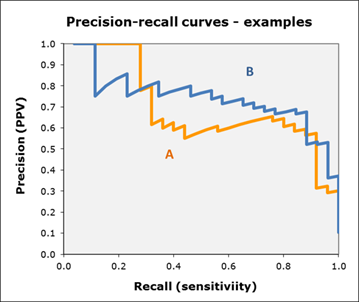FIG. 1: Precision-recall curves – examples

Precision-recall curves are often zigzag curves frequently going up and down. Therefore, precision-recall curves tend to cross each other much more frequently than ROC curves. This can make comparisons between curves challenging. However, curves close to the PRC for a perfect test (see later) have a better performance level than the ones closes to the baseline. In other words, a curve above the other curve has a better performance level.

### The difference between ROC curves and precision-recall curves

The main difference between ROC curves and precision-recall curves is that the number of true-negative results is not used for making a PRC.

 Curve x-axis y-axis Concept Calculation Concept Calculation Precision-recall Recall TP / (TP + FN) Precision TP / (TP + FP) ROC 1-specificity FP / (FP + TN) Sensitivity TP / (TP + FN)

TABLE IV: Precision-recall curves vs. ROC curves

### The perfect test

The perfect test has no overlap of results for persons with and without disease, respectively. The perfect test is thus able to discriminate between persons with and without disease with 100 % sensitivity (= recall), 100 % specificity and 100 % precision (= positive predictive value).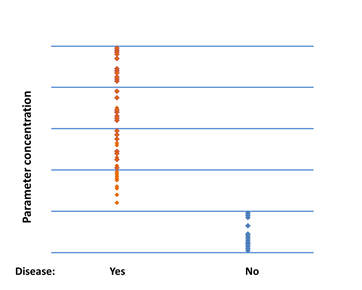FIG. 2: No overlap between persons with and without disease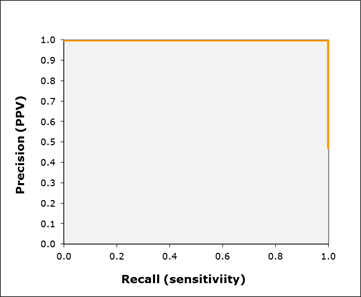FIG. 3: Precision-recall curve for a test with no overlap between persons with and without disease

The perfect test will have a PRC that passes through the upper right corner (corresponding to 100 % precision and 100 % recall). Generally you can say that the closer a PRC is to the upper right corner, the better the test is.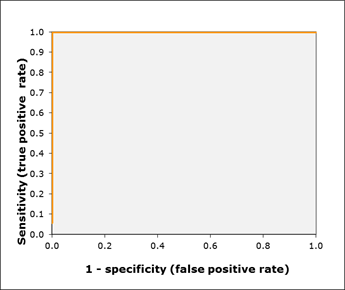FIG. 4: ROC curve for a test with no overlap between persons with and without disease

The perfect test will have a ROC curve that passes through the upper left corner (corresponding to 100 % sensitivity and 100 % specificity). Generally you can say that the closer a ROC curve is to the upper left corner, the better the test is.

### The worthless test

When we have a complete overlap between the results from persons with and without disease, we have a worthless test. A worthless test has a discriminating ability equal to flipping a coin.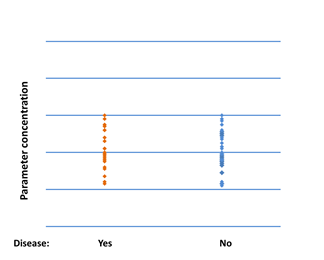FIG. 5: Complete overlap between persons with and without disease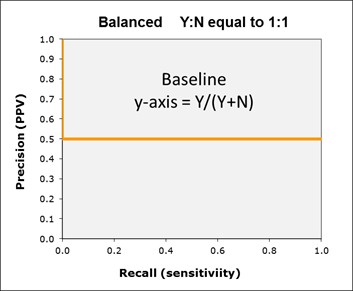FIG. 6: Precision-recall curve for a test with complete overlap of results between persons with and without disease – balanced distribution Y:N equal to 1:1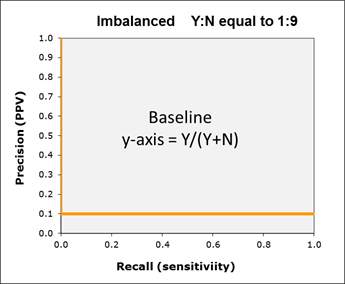FIG. 7: Precision-recall curve for a test with complete overlap of results between persons with and without disease – imbalanced distribution Y:N equal to 1:9

A PCR plot for a test with complete overlap of results between persons with and without disease will be determined by the ratio between the two groups:

For the balanced data set with Y:N equal to 1:1, you will due to the complete overlap of data for each cut-off have the same number of persons with disease as persons without disease. If X is identical to the number of persons with disease, the PPV will be X / (X + X), which is equal to 0.5.

For the imbalanced data set with Y:N equal to 1:9, you will due to the complete overlap of data for each cut-off have nine persons without disease for every person with disease. If X is identical to the number of persons with disease, the PPV will be X / (X + 9 × X), which is equal to 0.1.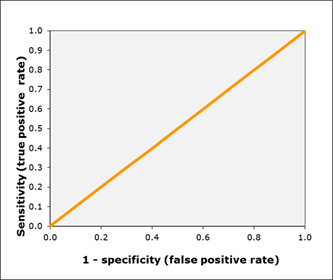FIG. 8: ROC curve for a test with complete overlap of results between persons with and without disease

A worthless test will have a ROC curve that falls on the diagonal line. It includes the point with 50 % sensitivity and 50 % specificity. The ratio of persons with and without disease will not have an impact on the ROC curve.

### Why precision-recall curves are better than ROC curves in imbalanced populations

In the following we will look at the hypothetical parameter C. We will look at a balanced data set and at an imbalanced data set. The balanced data set contains 80 results from persons with disease and 80 results from persons without disease. The imbalanced data set contains all the same individual results as the balanced data set and in addition it contains 640 results from persons without disease.

All the additional 640 results are below the lowest sample in the balanced data set. In Fig. 9 the difference is illustrated by the box with the additional 640 results.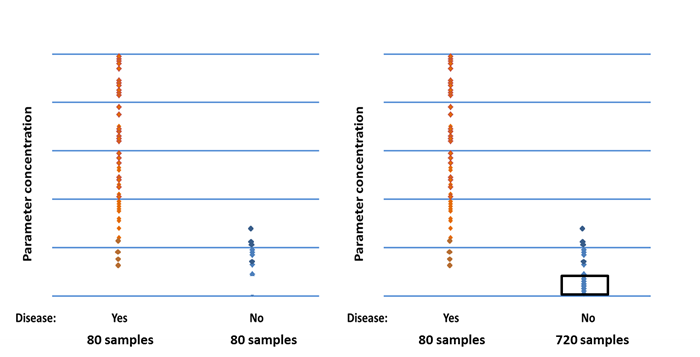FIG. 9: Balanced (left) vs. imbalanced (right) data set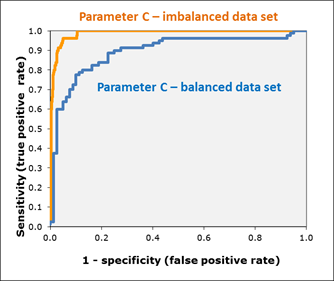FIG. 10: Balanced vs. imbalanced data set – ROC curves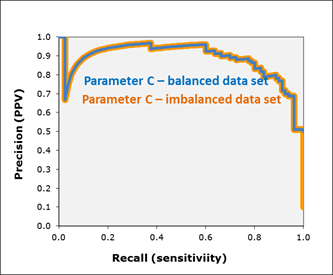FIG. 11: Balanced vs. imbalanced data set – precision-recall curves

The imbalanced data set gives a much better ROC curve (closer to the upper left corner) compared to the balanced data set even though the imbalanced data set contains all the same individual results as the balanced data set, and in addition it contains 640 results from persons without disease and all these additional 640 results are below the lowest sample in the balanced data set.

However, if you look at the precision-recall curves, the two curves are completely overlapping. The only difference is that at recall (sensitivity) = 1, the precision (PPV) drops to 0.1 for the imbalanced data set, while it is 0.5 for the balanced data set.
The general assumption has so far been that if you compared the area under ROC curves for two tests, you would see the real differences in diagnostic performances.

You may ask yourself what you would like to know about a test. For diagnostic tests you would first of all like to know the sensitivity (= recall) because you want to be sure that the test identifies the vast majority of persons with a disease as having the disease.

Then you would like to know the positive predictive value (= precision) because the PPV tells you how sure you can be, when you have a positive result, that the person actually has the disease. Thus you know how good the test is at discriminating persons with disease from those without disease.

The example presented above clearly shows that an imbalanced data set with a large fraction of persons without disease in the test population will make a ROC curve look better than it would in a balanced data set with fewer persons without disease.

This calls for caution when comparing ROC curves for different parameters between studies. The example also shows that precision-recall curves are not impacted by imbalanced data sets and thus their use should be encouraged as a supplement to the routinely used ROC curves.

### Conclusion

Saito and Rehmsmeier (2015)  found in a literature analysis that ROC curves are very popular, their popularity has been increasing for the last decade, and they are the most widely used evaluation method with imbalanced data.

Based on their models for how to compare performance the authors conclude that changing the main evaluation method from ROC to PRC may influence many studies. That this is the case has been shown here.

The graphs presented for the hypothetical data sets above clearly demonstrate that adding a lot of patients without disease and with low test results to a study may improve the ROC curve significantly without any improvement in sensitivity or in positive predictive value of the parameter evaluated.

The precision-recall curves were not impacted by the addition of patients without disease and with low test results. Thus it can be highly recommended to use PRC as a supplement to the routinely used ROC curves to get the full picture when evaluating and comparing tests.

 Fraction of persons without disease who get a negative test result with the assay in question. Calculated as TN / (TN + FP).
 Fraction of persons without disease who get a negative test result with the assay in question. Calculated as TN / (TN + FP).

References
1. Saito T, Rehmsmeier M. The precision-recall plot is more informative than the ROC plot when evaluating binary classifiers on imbalanced datasets. PLoS One 2015; 10,3: e0118432

References
1. Saito T, Rehmsmeier M. The precision-recall plot is more informative than the ROC plot when evaluating binary classifiers on imbalanced datasets. PLoS One 2015; 10,3: e0118432Suzanne Ekelund

Principal Specialist, Clinical Biochemist, MSc
Denmark

Articles by this author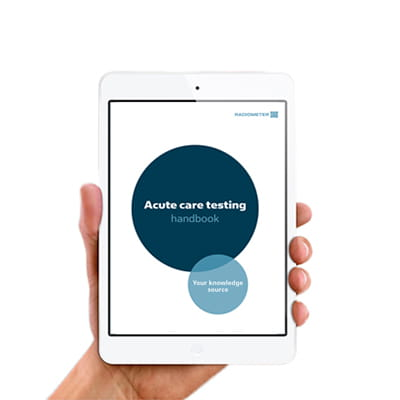## Get the acute care testing handbook

Your practical guide to critical parameters in acute care testing.